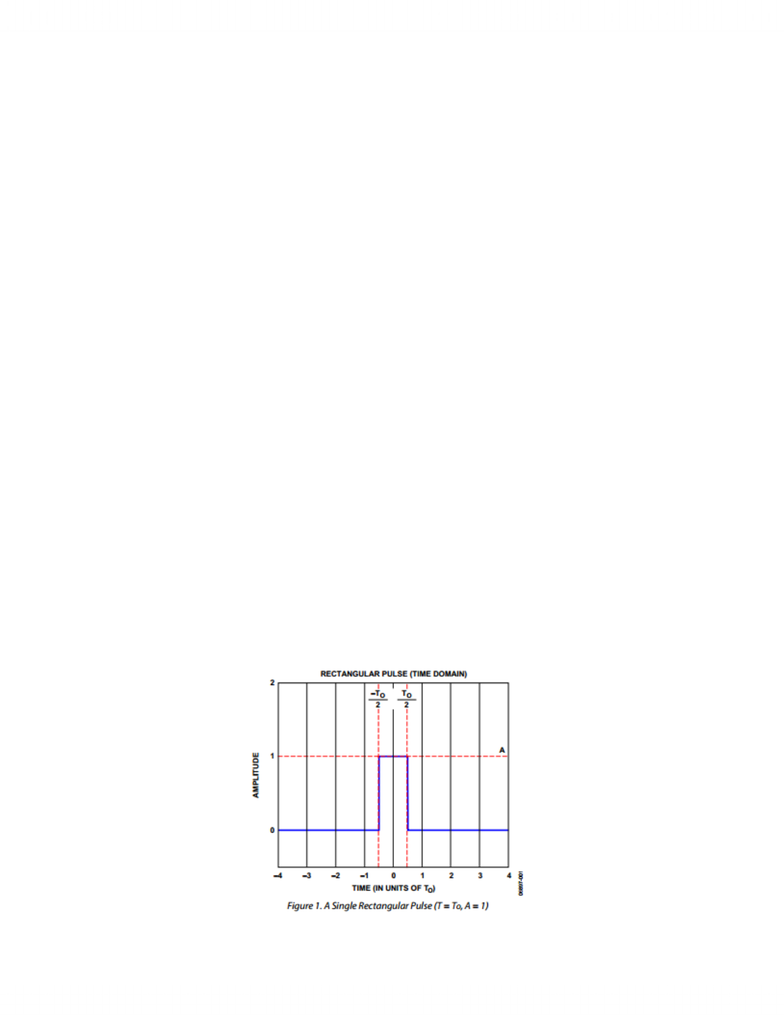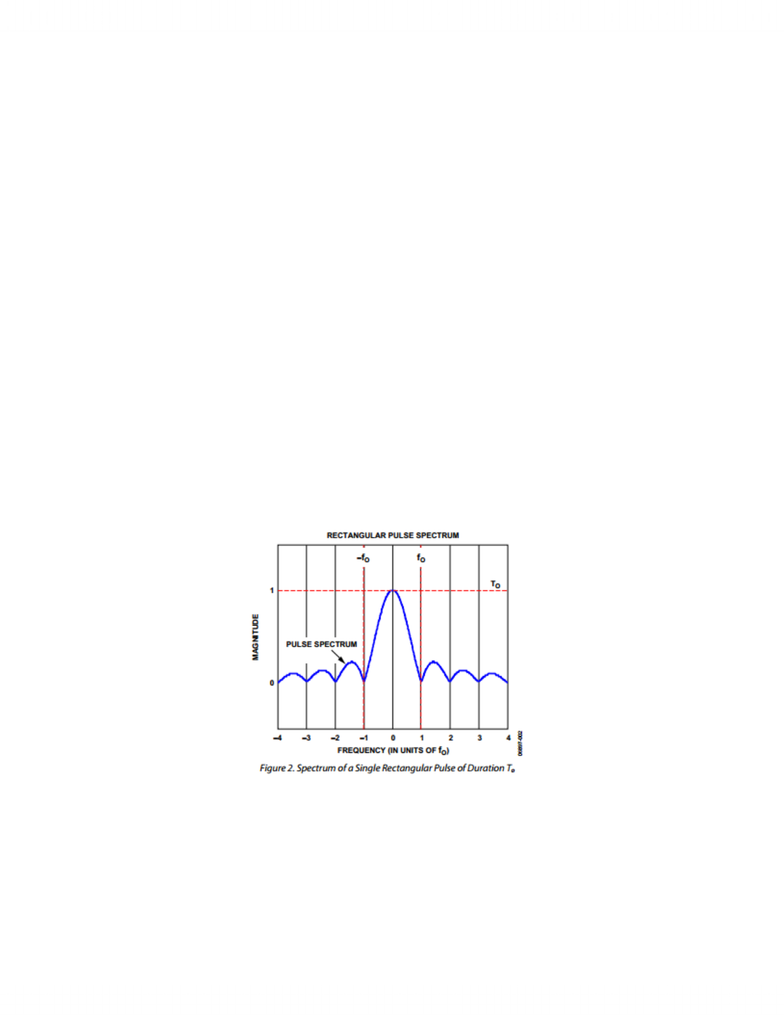Class Notes (1,000,000)
CA (620,000)
Queen's (10,000)
ELEC (30)
Lecture

ELEC 486 Lecture Notes - Wavelength-Division Multiplexing, Pulse Shaping, Sinc Filter

Department
Electrical and Computer Engineering Courses
Course Code
ELEC 486
Professor
Paul Cartledge

This preview shows pages 1-3. to view the full 12 pages of the document.Pulse/Spectrum Shaping
by
Christian Mastromattei, Jimmy Zhan
A report submitted to the Department of Electrical and Computer Engineering in conformity
with the requirements of ELEC 486
Faculty of Applied Science and Engineering
Queen’s University
April 16, 2013

Only pages 1-3 are available for preview. Some parts have been intentionally blurred.Introduction:
Bandwidth (BW) is one of the most crucial resources in the communication industry. Fiber optic
communication is band limited and typically occurs over the C-band. As modulation rates for
optical signals increase each channel occupies more BW in the optical band, meaning less
channels will be available at higher modulation rates. In order to squeeze more channels together
over a band, pulse shaping can be done. Pulse shaping is a process by which an optical signal is
shaped such that its BW is reduced in the frequency domain. Various techniques for pulse
shaping are used, with the objective of limiting the bandwidth of each channel while eliminating
Intersymbol Interference (ISI) and crosstalk.
The Rectangular Pulse:
The rectangular pulse is the most basic information unit in a digital transmission scheme. It has a
defined amplitude and period. A sequence of such pulses constitutes the transmission of
information. The information is encoded in the amplitude of the pulse. The simplest case is when
a binary 0 is encoded as the absence of a pulse (A = 0) and a binary 1 is encoded as the presence
of a pulse (A = constant). Since each pulse spans the period T, the maximum pulse rate is 1/T
Hz. The pulse amplitude can be more sophisticated and take on multiple discrete levels
(including negative values), so that each pulse can represent more than one bit 

Only pages 1-3 are available for preview. Some parts have been intentionally blurred.Figure. A rectangular pulse, with period T0, and amplitude A.
In these cases where multiple amplitudes and/or multiple simultaneous pulses transmit a single
unit of data, each unit of data (or symbol) represent multiple bits. The group of bits that a single
unit of data represents is defined as symbol. The unit symbols per second, is defined as the baud
rate. The data transmission bits rate is the baud rate multiplied by the number of bits represented
by each symbol . This means that a lower transmission rate can be used to transmit symbols as
opposed to directly transmitting bits, which is the primary reason that the more sophisticated data
transmission systems .
Spectrum of a Rectangular Pulse:
The Fourier Transform (spectrum) of the rectangular pulse is a sinc function, which is sin(x)/x.
This result is the same for any amplitude or frequency of the rectangular pulse. Although the
amplitude of the rectangular pulse does proportionally affect the magnitude of the frequency
response peaks.
Figure. The frequency spectrum of a rectangular pulse, note the null points at integer multiples of
f0.
The sinc function is characterized by these peaks extending from negative infinity to positive
infinity, but an important observation is that the null points (where the spectral magnitude is